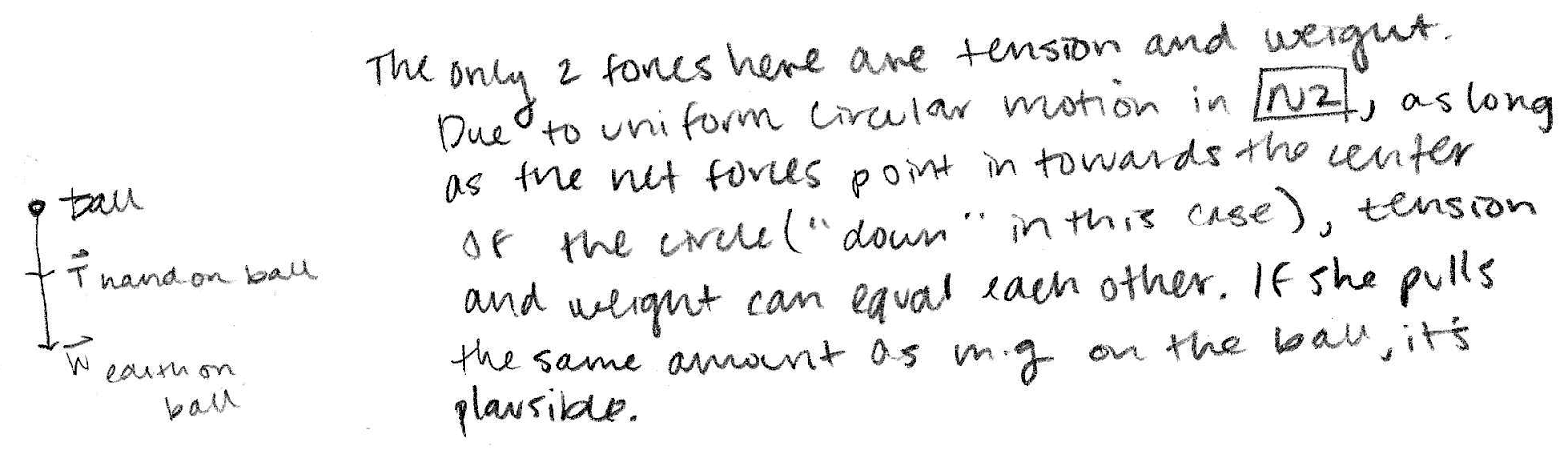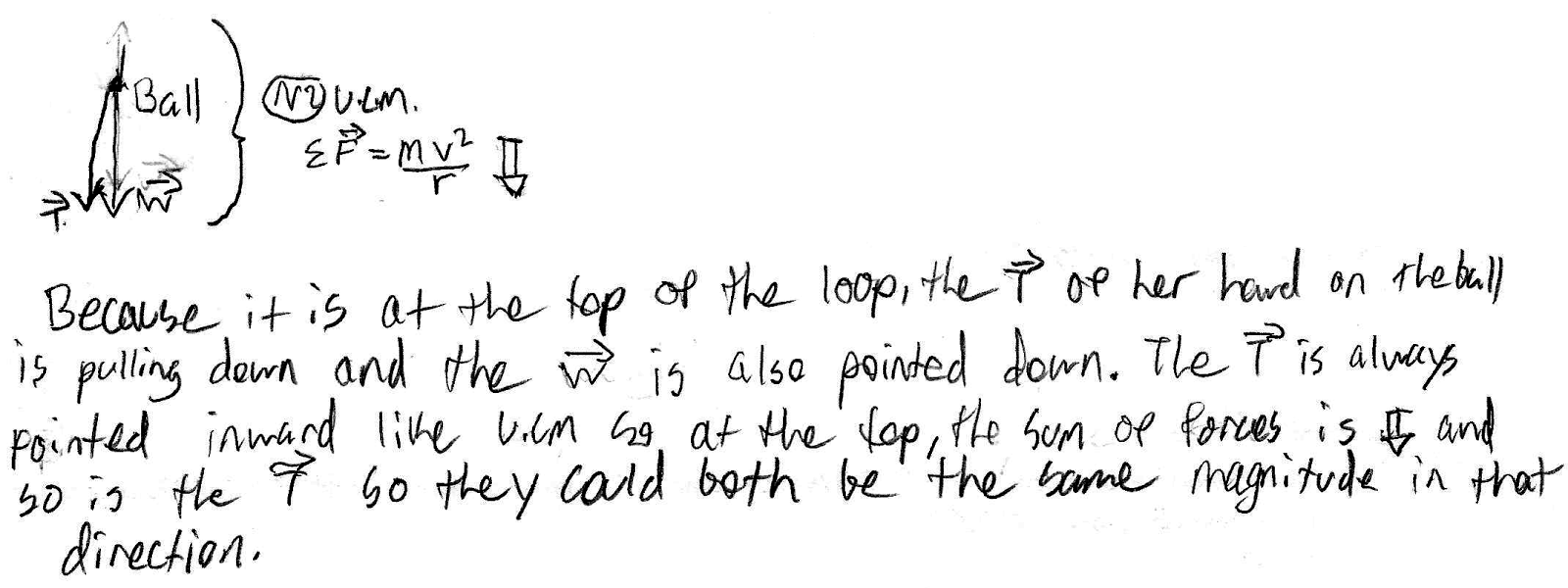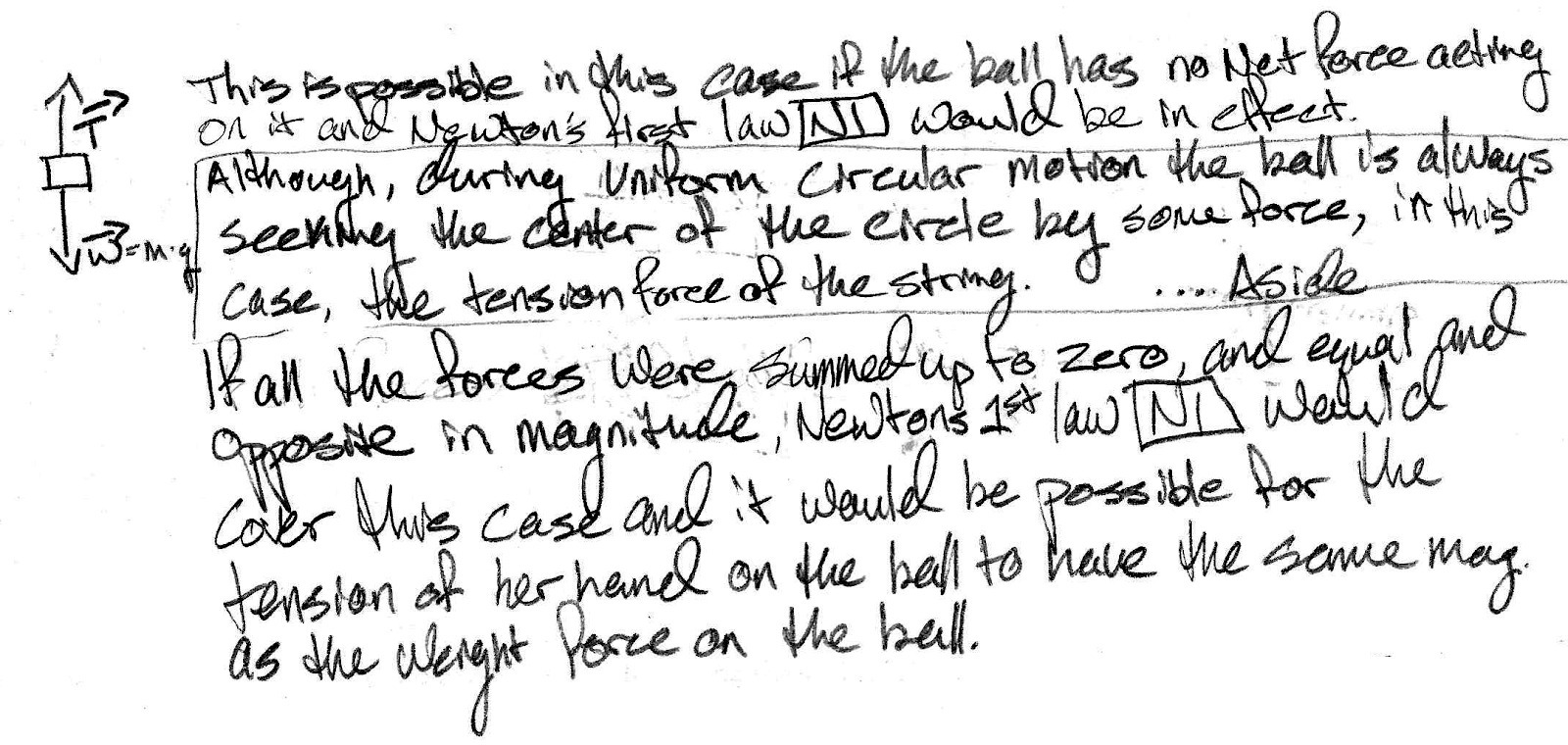## 20171020

### Physics midterm question: forces on string-twirled ball

Physics 205A Midterm 1, fall semester 2017
Cuesta College, San Luis Obispo, CAA Physics 205A student twirls a ball attached to a string in a vertical circle with constant speed. Discuss why it is possible for the tension force of her hand on the ball to have the same magnitude as the weight force of Earth on the ball, when the ball is swinging through the highest part of the circle. Explain your reasoning by using free-body diagram(s), the properties of forces and Newton's laws.

• p:
Correct. Complete free-body diagram, and discusses/demonstrates:
1. Newton's second law for uniform circular motion applies, such that while the ball is swinging through the highest part of the circle, the net force ΣF (of magnitude mv2/r) must point in towards the center of the circular motion--which is vertically downwards; and
2. while the ball is swinging through the highest part of the circle, there are two forces acting on the ball:
weight force w of Earth on the ball (mg, downwards);
tension force T of hand on the ball (downwards),
3. since both these two downwards forces add together to get the downwards net force, it is possible that they each have the same magnitude of one-half of mv2/r (for certain values of mass m, speed v, and radius r).
• r:
As (p), but argument indirectly, weakly, or only by definition supports the statement to be proven, or has minor inconsistencies or loopholes. Two of the three points (1)-(3) correct/complete.
• t:
Nearly correct, but argument has conceptual errors, or is incomplete. May argue T (upwards) = w (downwards) because of Newton's first law; or may draw T and w as both acting downwards but does not explicitly relate how Newton's second law requires a downwards net force that is met by their addition, and/or introduces normal forces, Newton's third law, etc.
• v:
Limited relevant discussion of supporting evidence of at least some merit, but in an inconsistent or unclear manner. Some substantive attempt at applying Newton's laws to free-body diagram.
• x:
Implementation of ideas, but credit given for effort rather than merit. No systematic application of Newton's laws to the forces on free-body diagram.
• y:
Irrelevant discussion/effectively blank.
• z:
Blank.
Sections 70854, 70855
Exam code: midterm01mOoL
p: 10 students
r: 7 students
t: 34 students
v: 11 students
x: 2 students
y: 0 students
z: 0 students

A sample "p" response (from student 4632):Another sample "p" response (from student 8659):A sample "t" response (from student 2500):